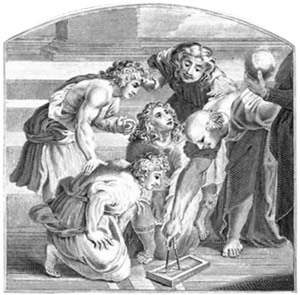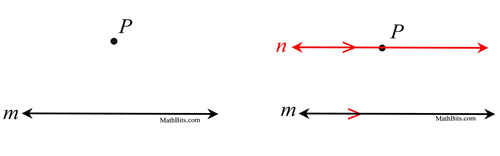The Parallel Postulate MathBitsNotebook.com Terms of Use   Contact Person: Donna RobertsHistorical reference:
Euclid (300 BC) was a Greek mathematician known as the "Father of Geometry". His works, some originating with earlier mathematicians, were published as the Elements (13 volumes devoted to Geometry) which is known as one of the most influential works in the history of mathematics.

In the Elements, Euclid created the foundation of Geometry using definitions, common notions, and his five famous postulates. (Postulates are statements made without proofs.) The fifth postulate deals with parallel lines.Euclid

 The Parallel Postulate In a plane, at most one line can be drawn through a point not on a given line parallel to the given line.From the time the fifth postulate was first stated, mathematicians believed that the statement was not a true postulate, but was rather a theorem which could be proven using the other postulates. It wasn't until the nineteenth century that mathematicians finally realized that it was not possible to "prove" the fifth postulate. While they could prove that the lines were parallel, they could not prove, using only existing postulates and theorems, that there was only ONE line through the point that was parallel to the given line. To this day, the Parallel Postulate is assumed true without proof.

The assumption that there possibly "could" be two lines through a point both parallel to a third line led to the discovery of non-Euclidean Geometries.